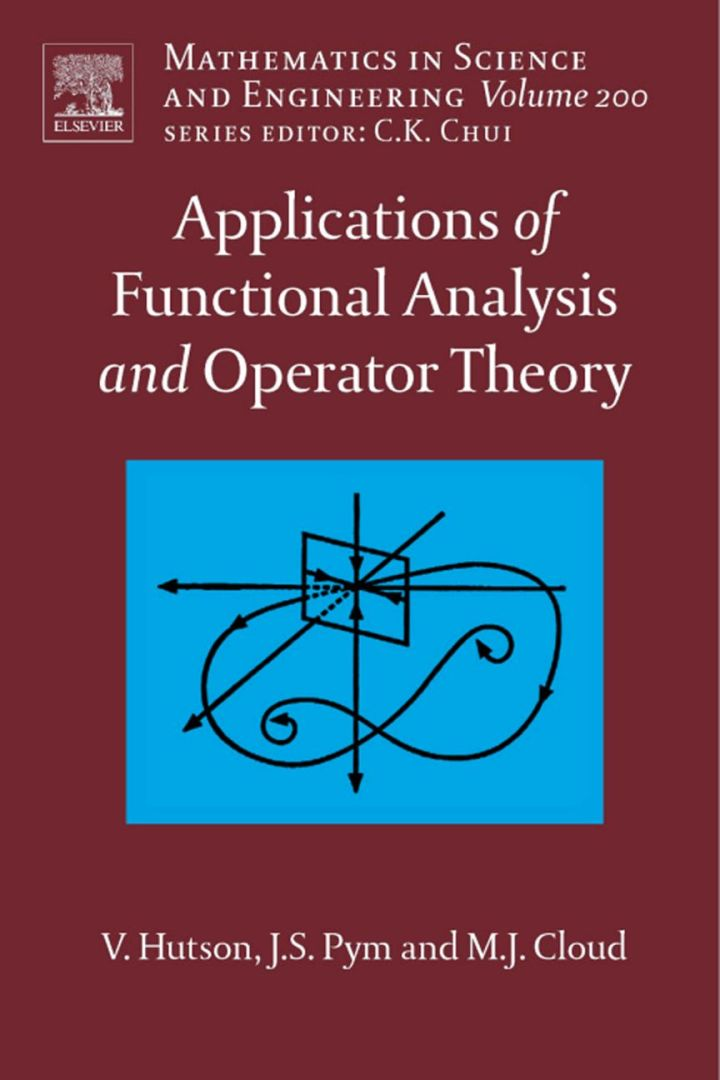# Applications of Functional Analysis and Operator Theory

## Applications of Functional Analysis and Operator Theory

By Hutson, V.; Pym, J.; Cloud, M.

### Rent or Buy eTextbook

Expires on Apr 21st, 2022
\$250

Publisher List Price: \$279.99
Savings: \$29.99

Functional analysis is a powerful tool when applied to mathematical problems arising from physical situations. The present book provides, by careful selection of material, a collection of concepts and techniques essential for the modern practitioner. Emphasis is placed on the solution of equations (including nonlinear and partial differential equations). The assumed background is limited to elementary real variable theory and finite-dimensional vector spaces.

Key Features

- Provides an ideal transition between introductory math courses and advanced graduate study in applied mathematics, the physical sciences, or engineering.
- Gives the reader a keen understanding of applied functional analysis, building progressively from simple background material to the deepest and most significant results.
- Introduces each new topic with a clear, concise explanation.
- Includes numerous examples linking fundamental principles with applications.
- Solidifies the reader’s understanding with numerous end-of-chapter problems.

· Provides an ideal transition between introductory math courses and advanced graduate study in applied mathematics, the physical sciences, or engineering.
· Gives the reader a keen understanding of applied functional analysis, building progressively from simple background material to the deepest and most significant results.
· Introduces each new topic with a clear, concise explanation.
· Includes numerous examples linking fundamental principles with applications.
· Solidifies the reader's understanding with numerous end-of-chapter problems.

Subject: Mathematics & Statistics -> Mathematics -> Mathematics General2nd edition
Publisher: Elsevier S & T 02/2005
Imprint: Elsevier Science
Language: English
Length: 432 pages

ISBN 10: 0444517901
ISBN 13: 9780444517906
Print ISBN: 9780444517906

Live Chats